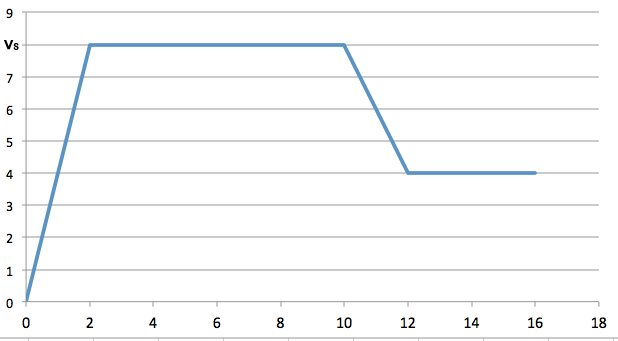# Velocity-time graphThe above is the velocity-time graph of a runner. How far does this runner travel for $16$ seconds?

The figure’s vertical scaling is set by $v_{s} = 8.0 \text{ m/s.}$

×